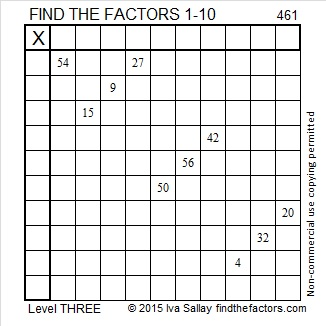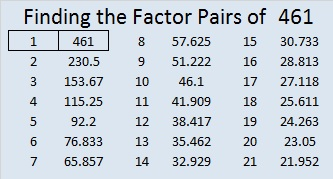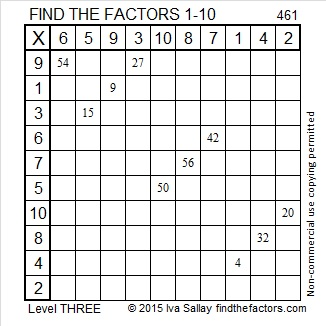# 461 and Level 3

461 = 19² + 10², and it is the hypotenuse in this primitive Pythagorean triple: 261-380-461.Print the puzzles or type the solution on this excel file:  10 Factors 2015-04-13

—————————————————————————————————

• 461 is a prime number.
• Prime factorization: 461 is prime.
• The exponent of prime number 461 is 1. Adding 1 to that exponent we get (1 + 1) = 2. Therefore 461 has exactly 2 factors.
• Factors of 461: 1, 461
• Factor pairs: 461 = 1 x 461
• 461 has no square factors that allow its square root to be simplified. √461 ≈ 21.4709How do we know that 461 is a prime number? If 461 were not a prime number, then it would be divisible by at least one prime number less than or equal to √461 ≈ 21.4709. Since 461 cannot be divided evenly by 2, 3, 5, 7, 11, 13, 17, or 19, we know that 461 is a prime number.

—————————————————————————————————

A Logical Approach to solve a FIND THE FACTORS puzzle: Find the column or row with two clues and find their common factor. Write the corresponding factors in the factor column (1st column) and factor row (top row).  Because this is a level three puzzle, you have now written a factor at the top of the factor column. Continue to work from the top of the factor column to the bottom, finding factors and filling in the factor column and the factor row one cell at a time as you go.